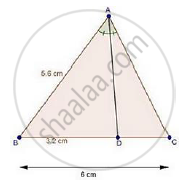Share

Books Shortlist
Your shortlist is empty

# Solution for In a δAbc, Ad is the Bisector of ∠A, Meeting Side Bc at D. If Ad = 5.6 Cm, Bc = 6cm and Bd = 3.2 Cm, Find Ac. - CBSE Class 10 - Mathematics

#### Question

In a ΔABC, AD is the bisector of ∠A, meeting side BC at D.

If AD = 5.6 cm, BC = 6cm and BD = 3.2 cm, find AC.

#### SolutionWe have,

In ΔABC, AD is the bisector of ∠A.

We know that, the internal bisector of an angle of a triangle divides the opposite side internally in the ratio of the containing the angle.

therefore"AB"/"AC"="BD"/"DC"

5.6/"AC"=3.2/(6-3.2)     [∵ DC = BC – BD]

rArr5.6/"AC"=3.2/2.8

rArr"AC"=(5.6xx2.8)/3.2

=(5.6xx7)/8=0.7xx7

= 4.9 cm

Is there an error in this question or solution?

#### Video TutorialsVIEW ALL 

Solution for question: In a δAbc, Ad is the Bisector of ∠A, Meeting Side Bc at D. If Ad = 5.6 Cm, Bc = 6cm and Bd = 3.2 Cm, Find Ac. concept: Angle Bisector. For the course CBSE
S